Practical Introduction to Continuous Wavelet Analysis

This example shows how to perform and interpret continuous wavelet analysis. The example helps you answer common questions such as: What is the difference between continuous and discrete wavelet analysis? Why are the frequencies, or scales in continuous wavelet analysis logarithmically spaced? In what types of signal analysis problems are continuous wavelet techniques particularly useful?

Continuous versus Discrete Wavelet Analysis

One question that people often have is: What is continuous about continuous wavelet analysis? After all, you are presumably interested in doing wavelet analysis in a computer and not with pencil and paper, and in a computer, nothing is really continuous. When the term continuous wavelet analysis is used in a scientific computing setting, it means a wavelet analysis technique with more than one wavelet per octave, or doubling of frequency, and where the shift between wavelets in time is one sample. This provides the resulting continuous wavelet transform (CWT) has two properties that are very useful in applications:

• The frequency content of a signal is more finely captured than with discrete wavelet techniques.

• The CWT has the same time resolution as the original data in each frequency band.

Additionally, in most applications of the CWT, it is useful to have complex-valued wavelets as opposed to real-valued wavelets. The main reason for this is that complex-valued wavelets contain phase information. See Compare Time-Varying Frequency Content in Two Signals for an example where phase information is useful. The examples in this tutorial use complex-valued wavelets exclusively.

See  for a detailed treatment of wavelet signal processing including continuous wavelet analysis with complex-valued wavelets.

Filters or Voices Per Octave

A term commonly used to designate the number of wavelet filters per octave is voices per octave. To illustrate this, construct a default continuous wavelet filter bank.

fb = cwtfilterbank
fb =
cwtfilterbank with properties:

VoicesPerOctave: 10
Wavelet: 'Morse'
SamplingFrequency: 1
SamplingPeriod: []
PeriodLimits: []
SignalLength: 1024
FrequencyLimits: []
TimeBandwidth: 60
WaveletParameters: []
Boundary: 'reflection'

If you examine the properties of the filter bank, by default there are 10 wavelet filters per octave (or VoicesPerOctave). Plot the frequency responses of the wavelet filters.

freqz(fb)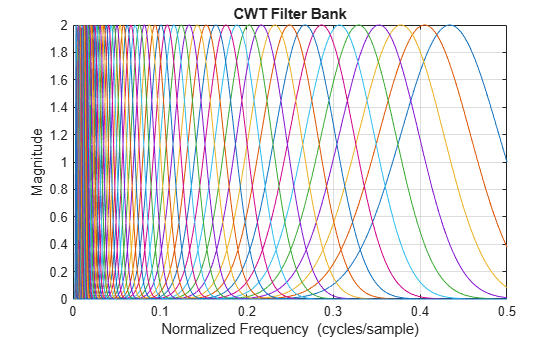The wavelet filters in a continuous analysis share the important constant-Q property with all wavelet filters, namely that their spread in frequency, or bandwidth, is proportional to their center frequency. In other words, wavelet filters are broader at higher frequencies than they are at lower frequencies. Because of the reciprocal relationship between time and frequency, this means that higher frequency wavelets are better localized in time (have smaller time support) than lower frequency wavelets. To see this, extract the center frequencies and time-domain wavelets from the filter bank. Plot the real parts of two wavelets, one high-frequency and one low frequency, for comparison.

psi = wavelets(fb);
F = centerFrequencies(fb);
figure
plot(real(psi(9,:)))
ylabel('Amplitude')
yyaxis right
plot(real(psi(end-16,:)))
ylabel('Amplitude')
axis tight
S1 = 'Wavelets With Center Frequencies';
S2 = [num2str(F(9),'%1.2f') ' and ' num2str(F(end-16),'%1.2f') ' cycles/sample'];
title({S1 ; S2})
xlabel('Sample')
legend('0.25 c/s Wavelet','0.01 c/s Wavelet')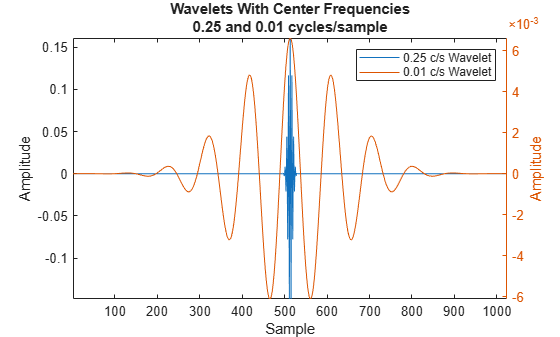In the frequency responses plot, the center frequencies do not exceed 0.45 cycles/sample. Find how many wavelet filters the filter bank has in one octave, or doubling of frequency. Confirm the number is equal to VoicesPerOctave.

numFilters10 = nnz(F >= 0.2 & F<= 0.4)
numFilters10 = 10

You can specify a different number of filters when you create the filter bank. Create a filter bank with 8 voices per octave and plot the frequency responses.

fb = cwtfilterbank('VoicesPerOctave',8);
figure
freqz(fb)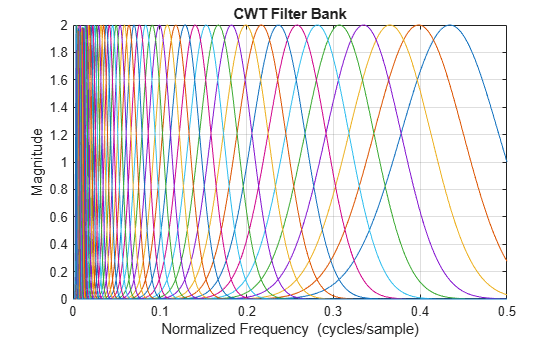Confirm that there are eight filters per octave in the filter bank.

F = centerFrequencies(fb);
numFilters8 = nnz(F >= 0.2 & F<= 0.4)
numFilters8 = 8

You can read a more detailed explanation of the differences between continuous and discrete wavelet analysis at Continuous and Discrete Wavelet Transforms.

Logarithmically Spaced Center Frequencies

One aspect of wavelet analysis that people can find a bit confusing is the logarithmic spacing of the filters.

Plot the center frequencies for the wavelet filter bank.

subplot(2,1,1)
plot(F)
ylabel('Cycles/Sample')
title('Wavelet Center Frequencies')
grid on
subplot(2,1,2)
semilogy(F)
grid on
ylabel('Cycles/Sample')
title('Semi-Log Scale')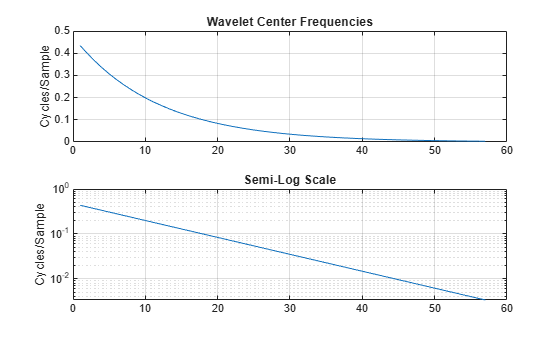The plots show that the wavelet center frequencies are not linearly spaced as is commonly the case with other filter banks. Specifically, the center frequencies are exponentially decreasing, so that the step size between higher center frequencies is larger than the step size between lower center frequencies. Why does this make sense for wavelets? Recall that wavelets are constant-Q filters, which means their bandwidths are proportional to their center frequencies. If you have a filter bank where every filter has the same bandwidth, like in the short-time Fourier transform, or spectrogram, filter bank, then to maintain a constant frequency overlap between filters you need a constant step size. However, with wavelets, the step size should be proportional to frequency like the bandwidth. For continuous wavelet analysis, the most common spacing is the base 2^(1/NV), where NV is the number of filters per octave, raised to integer powers. For comparison, the spacing used exclusively in discrete wavelet analysis is the base 2 raised to integer powers.

See  for a thorough treatment of discrete wavelet analysis. The following table summarizes the main similarities and differences between discrete and continuous wavelet techniques. For discrete techniques, the names of representative algorithms in MATLAB are provided in parentheses.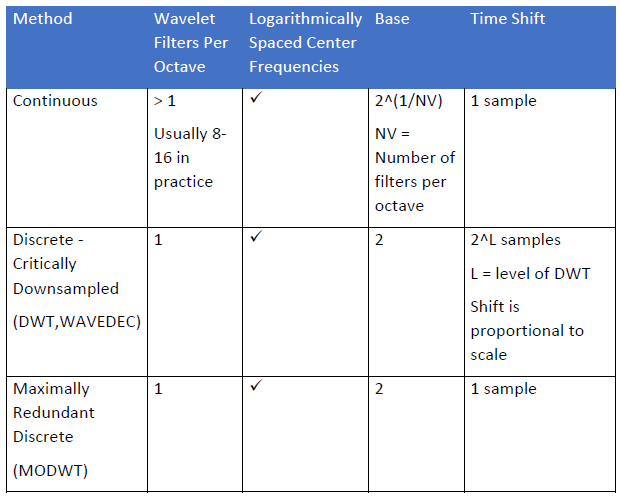Time-Frequency Analysis

Because wavelets are simultaneously localized in time and frequency, they are useful for a number of applications. For continuous wavelet analysis, the most common application area is time-frequency analysis. Furthermore, the following properties of the CWT make it particularly useful for certain classes of signals.

• The constant-Q property of the wavelet filters, which means higher frequency wavelets are shorter in duration and lower frequency wavelets are longer in duration.

• The one-sample time shift between wavelet filters in continuous analysis

To understand which classes of signals are good candidates for continuous wavelet analysis, consider a hyperbolic chirp signal.

figure
plot(t,hyperbolchirp)
axis tight
xlabel('Time (s)')
ylabel('Amplitude')
title('Hyperbolic Chirp')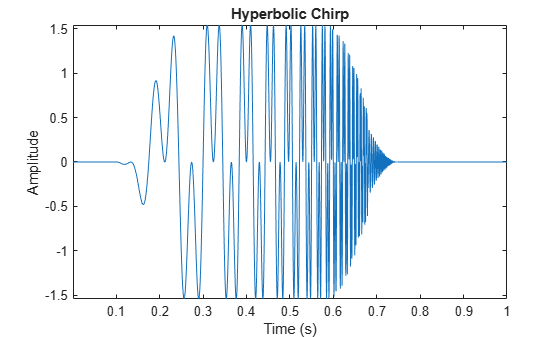The hyperbolic chirp is the sum of $\mathrm{sin}\left(\frac{15\pi }{0.8-t}\right)$ and $\mathrm{sin}\left(\frac{5\pi }{0.8-t}\right)$. The first component is active from 0.1 to 0.68 seconds, and the second component from 0.1 to 0.75 seconds. The frequency at each instant in time, or the instantaneous frequencies, of these components are $\frac{7.5}{\left(0.8-t{\right)}^{2}}$ and $\frac{2.5}{\left(0.8-t{\right)}^{2}}$ cycles/second respectively. This means that the instantaneous frequencies are very low near $t=0$ and increase rapidly as the time approaches 0.8 seconds.

Obtain the CWT of the chirp signal and plot the CWT along with the true instantaneous frequencies plotted as dashed lines.

Fs = 1/mean(diff(t));
[cfs,f] = cwt(hyperbolchirp,Fs);
helperHyperbolicChirpPlot(cfs,f,t)We see that the CWT shows a time-frequency representation that accurately captures the instantaneous frequencies of the hyperbolic chirp. Now analyze the same signal with the short-time Fourier transform which uses constant bandwidth filters. Use the default window size and overlap.

pspectrum(hyperbolchirp,2048,'spectrogram')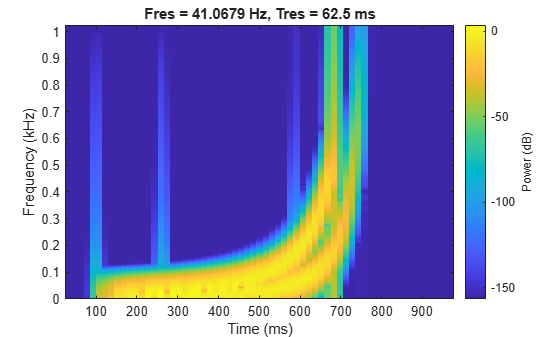Note that the spectrogram is unable to distinguish the two different instantaneous frequencies when the frequencies are low, or equivalently when the two instantaneous frequencies are close together. Based on the input length of 2048, the spectrogram is computed by default using a window length of 128 samples. This is equivalent to a time resolution of 62.5 msec given the sampling rate of 2048 Hz. Based on the default Kaiser window, this results in a frequency resolution of 41 Hz. This is too large to differentiate the instantaneous frequencies near t=0. What happens if we reduce the frequency resolution by approximately 1/2 in the hopes of better resolving the lower frequencies?

pspectrum(hyperbolchirp,2048,'spectrogram','FrequencyResolution',20, ...
'OverlapPercent',90)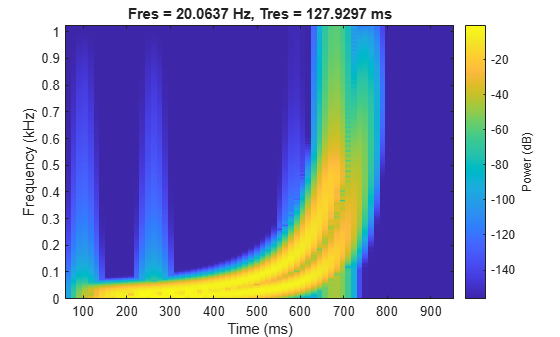The result is no better because any frequency separation we have gained at the lower frequencies results in the higher instantaneous frequencies smearing together in time. The spectrogram is a powerful time-frequency analysis technique, in fact arguably optimal in many applications, but like all techniques it has limitations. This particular type of signal presents a major challenge for fixed bandwidth filters. Ideally you want a long time response with narrow frequency support at low frequencies and a short time support with broader frequency support at high frequencies. The wavelet transform provides exactly that and is therefore quite successful in this case. An equivalent way to state this is that the wavelet transform has better time resolution at higher frequencies and better frequency resolution at lower frequencies.

In real-world signals, high-frequency events are often of short duration while lower frequency events are longer in duration. As an example, load and plot a human electrocardiogram (ECG) signal. The data is sampled at 180 Hz.

tm = 0:1/180:numel(wecg)*1/180-1/180;
plot(tm,wecg)
grid on
axis tight
title('Human ECG')
xlabel('Time (s)')
ylabel('Amplitude')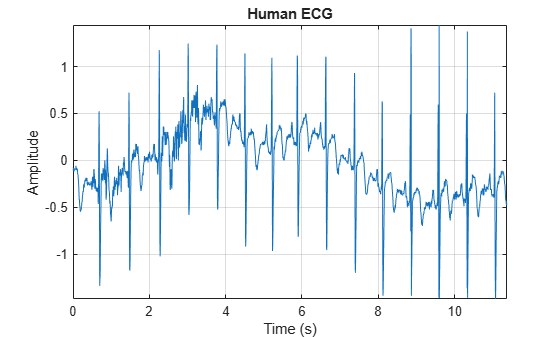The data consists of slowly varying components punctuated by high frequency transients, which represent the electrical impulse as it spreads through the heart. If we are interested in localizing these impulsive events accurately in time, we want the analysis window (filter) to be short. We are willing to sacrifice frequency resolution in this case for more accurate time localization. On the other hand, to identify the lower frequency oscillations, it is preferred to have a longer analysis window.

[cfs,f] = cwt(wecg,180);
imagesc(tm,f,abs(cfs))
xlabel('Time (s)')
ylabel('Frequency (Hz)')
axis xy
caxis([0.025 0.25])
title('CWT of ECG Data')The CWT shows nearly steady-state oscillations near 30 Hz and 37 Hz as well as the transient events denoting the heartbeats (QRS complexes). With this analysis, you are able to analyze both phenomena simultaneously in the same time-frequency representation.

As another example of high frequency transients punctuating slowly varying components, consider a time series of solar magnetic field magnitudes recorded hourly over the south pole of the sun by the Ulysses spacecraft from 21:00 UT on December 4, 1993 to 12:00 UT on May 24, 1994. See  pp. 218–220 for a complete description of this data.

plot(sm_dates,sm)
axis tight
title('Solar Magnetic Field Magnitudes')
xlabel('Date')
ylabel('Magnitude')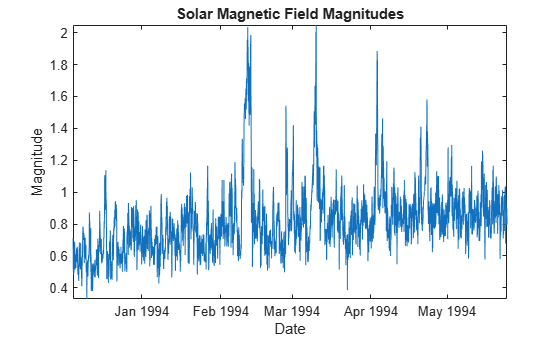The raw time data shows an overall roughly linear trend with several impulsive events, or shock waves. Shock waves are produced when a fast solar wind or fast coronal mass ejection overtakes a slow solar wind. In the time series, we see significant shock wave structures occurring approximately on the following dates: February 11, February 26, March 10, April 3, and April 23.

Perform a continuous wavelet analysis of the data and plot the CWT magnitudes with the time series data on the same plot.

helperSolarMFDataPlot(sm,sm_dates)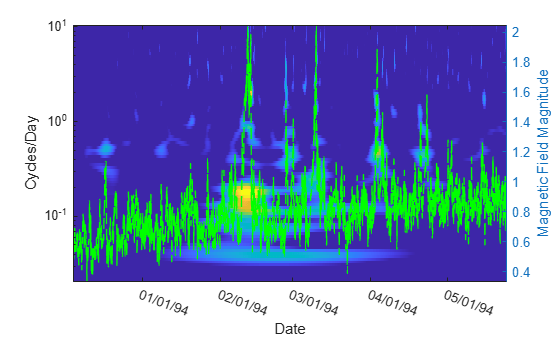The CWT captures the impulsive events at the same times they occur in the time series. However, the CWT also reveals lower frequency features of the data hidden in the time series. For example, starting before and extending beyond the shock wave structure on February 11, 1994, there is a low-frequency steady state event (near 0.04 cycles/day). This results from a coronal mass ejection (CME) event likely occurring in a different solar region which reached the Ulysses spacecraft at the same time as the closer shock wave.

With the ECG and solar magnetic field magnitude examples, we have two time series from very disparate mechanisms generating similar data: signals with both short-duration transients and longer duration low frequency oscillations. Characterizing both in the same time-frequency representation is advantageous.

Localize Transients

Transient events in time series data are often one of the most informative events. Transient events can indicate an abrupt change in the data-generating mechanism, which you would like to detect and localize in time. Examples include faults in machinery, problems with sensors, financial "shocks" in economic time series, and many others. As an example, consider the following signal.

rng default;
dt = 0.001;
t = 0:dt:1.5-dt;
x = cos(2*pi*150*t).*(t>=0.1 & t<0.5)+sin(2*pi*200*t).*(t>0.7 & t <= 1.2);
x([222 800]) = x([222 800 ])+[-2 2];
figure;
plot(t.*1000,x);
xlabel('Milliseconds'); ylabel('Amplitude');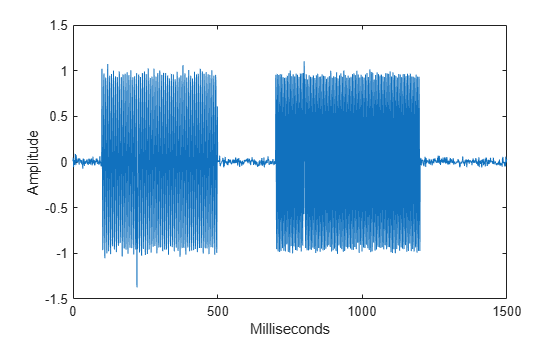The signal consists of two sinusoidal components that turn on and off abruptly with additional "defects" at 222 milliseconds and 800 milliseconds. Obtain the CWT of the signal and plot only the finest-scale, or highest center frequency, wavelet coefficients. Create a second axis and plot the original time data.

cfs = cwt(x,1000,'amor');
plot(t,abs(cfs(1,:)),'linewidth',2)
ylabel('Magnitude')
set(gca,'XTick',[0.1 0.222 0.5 0.7 0.8 1.2])
yyaxis right
plot(t,x,'--')
ylabel('Amplitude')
xlabel('Seconds')
hold off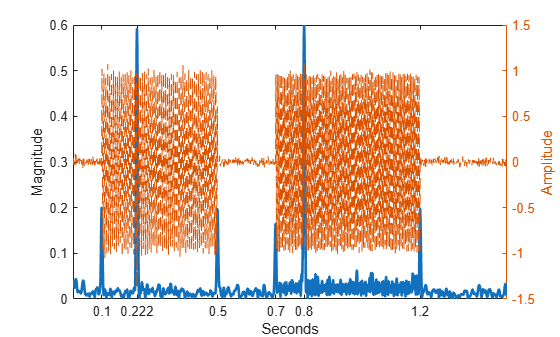The finest-scale CWT coefficients localize all the abrupt changes in the data. The CWT coefficients show peaks where the sinusoidal components turn on and off as well as localizing the defects in the sinusoids at 222 milliseconds and 800 milliseconds. Because the CWT coefficients have the same time resolution as the data, it is often useful to use the CWT fine-scale magnitudes in order to detect transient changes in the data. To most accurately localize these transients, use the finest-scale (highest frequency) coefficients.

Sharpen Time-Frequency Analysis

Any time-frequency transform that uses filters, like wavelets in the case of the CWT, or modulated windows in the case of the short-time Fourier transform, necessarily smears the picture of the signal in time and frequency. The uncertainty in the localization of the signal's energy in time and frequency comes from the spread of the filters in time and frequency. Synchrosqueezing is a technique that attempts to compensate for this smearing by "squeezing" the transform along the frequency axis. To illustrate this with wavelets, obtain the CWT of a signal consisting of an amplitude and frequency modulated (AM-FM) signal and an 18-Hz sinusoid. The AM-FM signal is defined by the equation

$\left(2+\mathrm{cos}\left(4\pi t\right)\right)\mathrm{sin}\left(2\pi 231t+90\mathrm{sin}\left(3\pi t\right)\right)$

sig = sig1+sig2;
cwt(sig,sampfreq,'amor')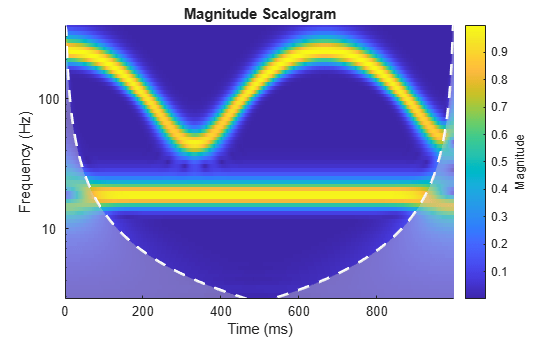While the CWT shows both the 18-Hz sine wave as well as the AM-FM component, we see that the 18-Hz sine wave certainly appears spread out in frequency. Now use synchrosqueezing to sharpen the frequency estimates.

[sst,f] = wsst(sig,sampfreq);
figure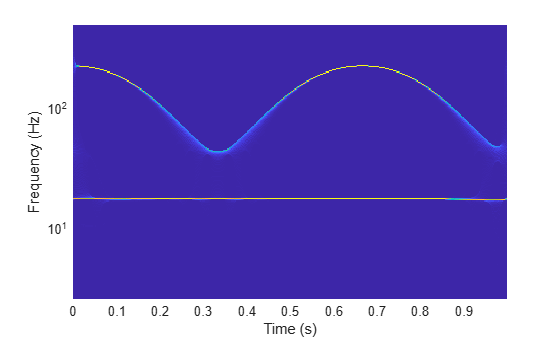The synchrosqueezed transform has largely compensated for the spread in time frequency introduced by the wavelet filters. Synchrosqueezing has squeezed the relatively broad peaks in time-frequency into narrow ridges. You can actually extract these ridges and reconstruct the individual components from the time-frequency ridges.

[fridge,iridge] = wsstridge(sst,5,f,'NumRidges',2);
figure;
contour(t,f,abs(sst));
grid on;
title('Synchrosqueezed Transform with Ridges');
xlabel('Time (secs)'); ylabel('Hz');
hold on;
plot(t,fridge,'k--','linewidth',2);
hold off;Finally reconstruct approximations to the components from the time-frequency ridges and compare the results to the original components.

xrec = iwsst(sst,iridge);
helperPlotComponents(xrec,sig1,sig2,t)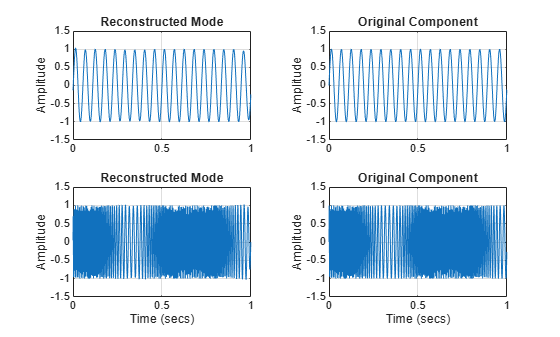Compare Time-Varying Frequency Content in Two Signals

Often you have two signals which may be related in some way. One signal may determine behavior in another, or the signals may simply be correlated because of some influence extrinsic to both signals. If you obtain the CWT of two signals, you can use those transforms to obtain the wavelet coherence, a measure of time-varying correlation.

Wavelet coherence for each time instant and center frequency produces a coherence value between 0 and 1 which quantifies the strength of the correlation between the two signals. Because complex-valued wavelets are used, there is also phase information, which can be used to infer the lead-lag relationship between the signals. As an example, consider the following two signals.

t = 0:0.001:2;
X = cos(2*pi*10*t).*(t>=0.5 & t<1.1)+ ...
cos(2*pi*50*t).*(t>= 0.2 & t< 1.4)+0.25*randn(size(t));
Y = sin(2*pi*10*t).*(t>=0.6 & t<1.2)+...
sin(2*pi*50*t).*(t>= 0.4 & t<1.6)+ 0.35*randn(size(t));
figure
subplot(2,1,1)
plot(t,X)
ylabel('Amplitude')
subplot(2,1,2)
plot(t,Y)
ylabel('Amplitude')
xlabel('Time (s)')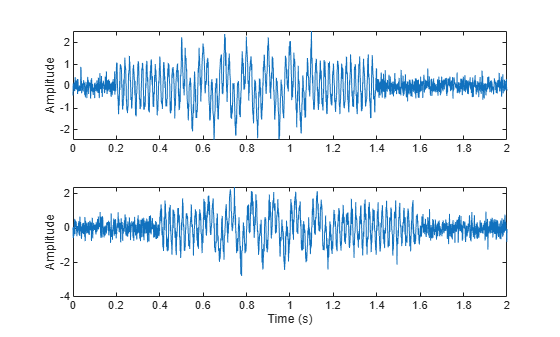Both signals consist two sine waves (10 Hz and 50 Hz) in white noise. The sine waves have slightly different time supports. Note that the 10 Hz and 50 Hz components in Y are lagged by 1/4 cycle with respect to the corresponding components in X. Plot the wavelet coherence of the two signals.

figure
wcoherence(X,Y,1000,'PhaseDisplayThreshold',0.7)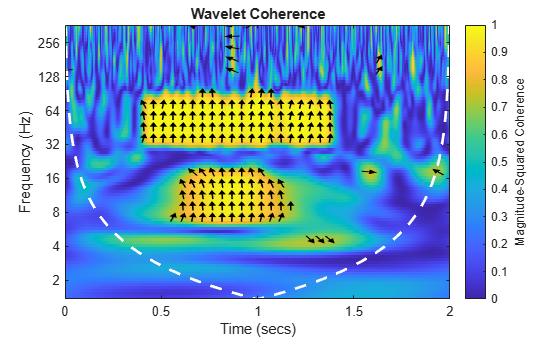The wavelet coherence estimate captures that the signals are highly correlated near 50 and 10 Hz and only during the correct time intervals. The dark arrows on the plot show the phase relationship between the signals. Here the arrows point straight up which indicates a 90-degree phase relationship between the two signals. That is exactly the relationship dictated by the cosine-sine terms in the signals.

For a real-world example, consider the El Nino Region 3 data and deseasonalized All Indian Rainfall Index from 1871 to late 2003. The data are sampled monthly. The Nino 3 time series is a record of monthly sea surface temperature anomalies in degrees Celsius recorded from the equatorial Pacific at longitudes 90 degrees west to 150 degrees west, and latitudes 5 degrees north to 5 degrees south. The All Indian Rainfall Index represents average Indian rainfalls in millimeters with seasonal components removed.

figure
subplot(2,1,1)
plot(datayear,nino)
title('El Nino Region 3 -- SST Anomalies')
ylabel('Degrees Celsius')
xlabel('Year')
axis tight
subplot(2,1,2)
plot(datayear,air)
axis tight
title('Deseasonalized All Indian Rainfall Index')
ylabel('Millimeters')
xlabel('Year')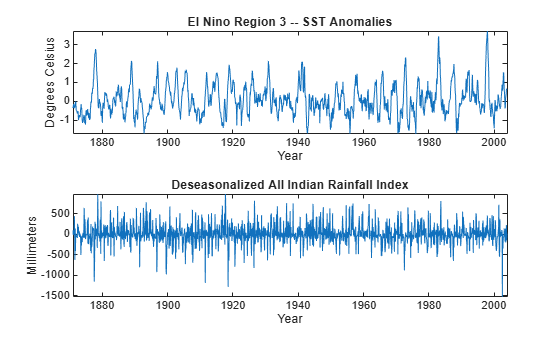Compute the wavelet coherence between the two time series. Set the phase display threshold to 0.7. To display the periods in years, specify the sampling interval as 1/12 of a year.

figure
wcoherence(nino,air,years(1/12),'PhaseDisplayThreshold',0.7);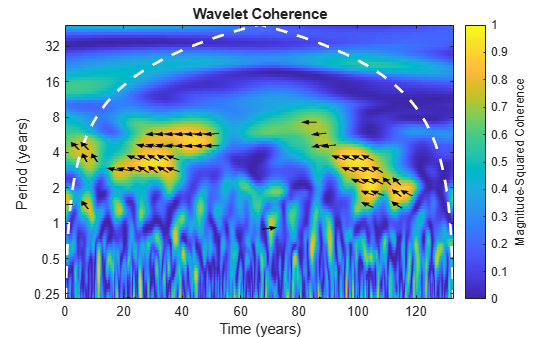The plot shows time-localized areas of strong coherence occurring in periods that correspond to the typical El Nino cycles of 2 to 7 years. The plot also shows that there is an approximate 3/8-to-1/2 cycle delay between the two time series at those periods. This indicates that periods of sea warming consistent with El Nino recorded off the coast of South America are correlated with rainfall amounts in India approximately 17,000 km away, but that this effect is delayed by approximately 1/2 a cycle (1 to 3.5 years).

Conclusions

In this example you learned:

• What differentiates continuous from discrete wavelet analysis.

• How the constant-Q and one-sample time shift properties of the CWT allow you to analyze very different signal structures simultaneously.

• A time-frequency reassignment technique using the CWT.

• How you can use the CWT to compare frequency content in two signals.

See Time-Frequency Analysis for many more highlighted topics and featured examples on continuous wavelet analysis.

References

 Mallat, S. G. A Wavelet Tour of Signal Processing: The Sparse Way. 3rd ed. Amsterdam ; Boston: Elsevier/Academic Press, 2009.

 Percival, Donald B., and Andrew T. Walden. Wavelet Methods for Time Series Analysis. Cambridge: Cambridge University Press, 2000. https://doi.org/10.1017/CBO9780511841040.

Wavelet Toolbox DocumentationGet trial now